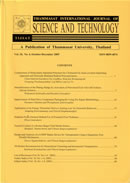THAMMASAT INTERNATIONAL JOURNAL OF SCIENCE & TECHNOLOGY

Volume 25, No. 01, Month JANUARY, Year 2020, Pages 1 - 10

A new family of distribution with application on two real datasets on survival problem

Kanak Modi, Devendra Kumar, Yudhveer Singh

Abstract

In this paper we introduce a new Modi family of continuous probability distributions with application on patients suffering from disease and their survival times. The proposed distribution possesses a density function with three parameters and an inverted J-shape hazard rate function. We studied the nature of proposed distribution with the help of its mathematical and statistical properties. The probability density function of order statistics for this distribution is also obtained. We perform classical estimation of parameters by using the technique of maximum likelihood estimate. We apply it to two real datasets and show that it provides better fit than other well known distributions.

Keywords

Exponential distribution, Maximum likelihood estimation, Order statistics, Probability weighted moments, Renyi entropy

THAMMASAT INTERNATIONAL JOURNAL OF SCIENCE & TECHNOLOGY